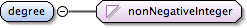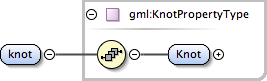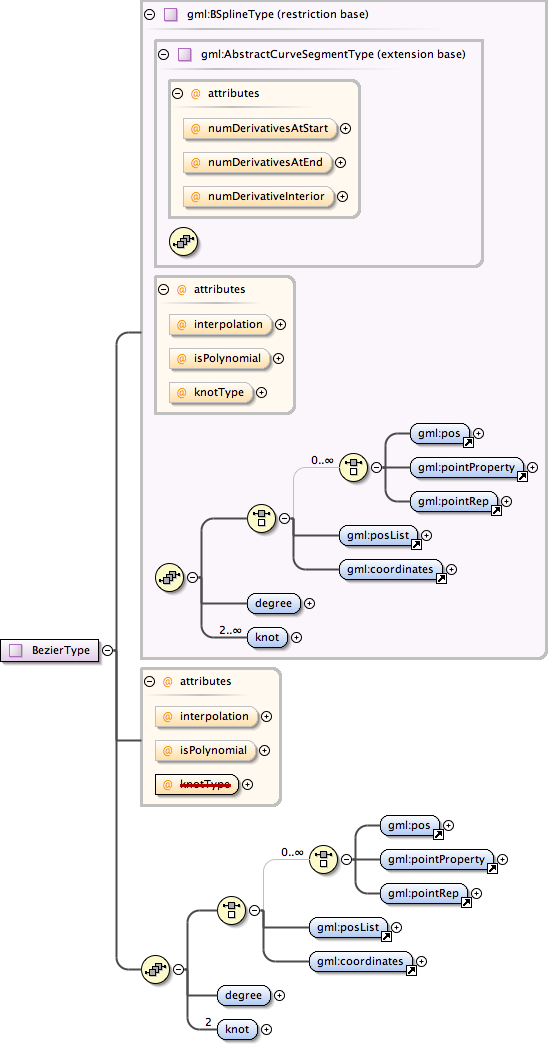### Showing:

 Annotations Attributes Diagrams Facets Instances Properties Source Used by
Element gml:BezierType / gml:degree
Namespace http://www.opengis.net/gml
Annotations
 `The attribute "degree" shall be the degree of the polynomial used for interpolation in this spline.`
DiagramType nonNegativeInteger
Properties
 content: simple
Source
 ``` The attribute "degree" shall be the degree of the polynomial used for interpolation in this spline. ```
Schema location http://schemas.opengis.net/gml/3.1.1/base/geometryPrimitives.xsd
Element gml:BezierType / gml:knot
Namespace http://www.opengis.net/gml
Annotations
 `The property "knot" shall be the sequence of distinct knots used to define the spline basis functions.`
DiagramType gml:KnotPropertyType
Properties
 content: complex minOccurs: 2 maxOccurs: 2
Model gml:Knot
Children gml:Knot
Instance
 ``` {1,1} ```
Source
 ``` The property "knot" shall be the sequence of distinct knots used to define the spline basis functions. ```
Schema location http://schemas.opengis.net/gml/3.1.1/base/geometryPrimitives.xsd
Complex Type gml:BezierType
Namespace http://www.opengis.net/gml
Annotations
 `Bezier curves are polynomial splines that use Bezier or Bernstein polynomials for interpolation purposes. It is a special case of the B-Spline curve with two knots.`
DiagramType restriction of gml:BSplineType
Type hierarchy
Used by
 Element gml:Bezier
Model (gml:pos | gml:pointProperty | gml:pointRep | gml:posList | gml:coordinates) , gml:degree , gml:knot{2,2}
Children gml:coordinates, gml:degree, gml:knot, gml:pointProperty, gml:pointRep, gml:pos, gml:posList
Attributes
QName Type Fixed Default Use Annotation
interpolation gml:CurveInterpolationType polynomialSpline optional
 ```The attribute "interpolation" specifies the curve interpolation mechanism used for this segment. This mechanism uses the control points and control parameters to determine the position of this curve segment. For a Bezier the interpolation is fixed as "polynomialSpline".```
isPolynomial boolean true optional
 `The attribute isPolynomial is set to true as this is a polynomial spline.`
numDerivativeInterior integer 0 optional
 ```The attribute "numDerivativesInterior" specifies the type of continuity that is guaranteed interior to the curve. The default value of "0" means simple continuity, which is a mandatory minimum level of continuity. This level is referred to as "C 0 " in mathematical texts. A value of 1 means that the function and its first derivative are continuous at the appropriate end point: "C 1 " continuity. A value of "n" for any integer means the function and its first n derivatives are continuous: "C n " continuity. NOTE: Use of these values is only appropriate when the basic curve definition is an underdetermined system. For example, line string segments cannot support continuity above C 0 , since there is no spare control parameter to adjust the incoming angle at the end points of the segment. Spline functions on the other hand often have extra degrees of freedom on end segments that allow them to adjust the values of the derivatives to support C 1 or higher continuity.```
numDerivativesAtEnd integer 0 optional
 ```The attribute "numDerivativesAtEnd" specifies the type of continuity between this curve segment and its successor. If this is the last curve segment in the curve, one of these values, as appropriate, is ignored. The default value of "0" means simple continuity, which is a mandatory minimum level of continuity. This level is referred to as "C 0 " in mathematical texts. A value of 1 means that the function and its first derivative are continuous at the appropriate end point: "C 1 " continuity. A value of "n" for any integer means the function and its first n derivatives are continuous: "C n " continuity. NOTE: Use of these values is only appropriate when the basic curve definition is an underdetermined system. For example, line string segments cannot support continuity above C 0 , since there is no spare control parameter to adjust the incoming angle at the end points of the segment. Spline functions on the other hand often have extra degrees of freedom on end segments that allow them to adjust the values of the derivatives to support C 1 or higher continuity.```
numDerivativesAtStart integer 0 optional
 ```The attribute "numDerivativesAtStart" specifies the type of continuity between this curve segment and its predecessor. If this is the first curve segment in the curve, one of these values, as appropriate, is ignored. The default value of "0" means simple continuity, which is a mandatory minimum level of continuity. This level is referred to as "C 0 " in mathematical texts. A value of 1 means that the function and its first derivative are continuous at the appropriate end point: "C 1 " continuity. A value of "n" for any integer means the function and its first n derivatives are continuous: "C n " continuity. NOTE: Use of these values is only appropriate when the basic curve definition is an underdetermined system. For example, line string segments cannot support continuity above C 0 , since there is no spare control parameter to adjust the incoming angle at the end points of the segment. Spline functions on the other hand often have extra degrees of freedom on end segments that allow them to adjust the values of the derivatives to support C 1 or higher continuity.```
Source
 ``` Bezier curves are polynomial splines that use Bezier or Bernstein polynomials for interpolation purposes. It is a special case of the B-Spline curve with two knots. GML supports two different ways to specify the control points of a curve segment. 1. A sequence of "pos" (DirectPositionType) or "pointProperty" (PointPropertyType) elements. "pos" elements are control points that are only part of this curve segment, "pointProperty" elements contain a point that may be referenced from other geometry elements or reference another point defined outside of this curve segment (reuse of existing points). 2. The "posList" element allows for a compact way to specifiy the coordinates of the control points, if all control points are in the same coordinate reference systems and belong to this curve segment only. Deprecated with GML version 3.1.0. Use "pointProperty" instead. Included for backwards compatibility with GML 3.0.0. Deprecated with GML version 3.1.0. Use "posList" instead. The attribute "degree" shall be the degree of the polynomial used for interpolation in this spline. The property "knot" shall be the sequence of distinct knots used to define the spline basis functions. The attribute "interpolation" specifies the curve interpolation mechanism used for this segment. This mechanism uses the control points and control parameters to determine the position of this curve segment. For a Bezier the interpolation is fixed as "polynomialSpline". The attribute isPolynomial is set to true as this is a polynomial spline. The property "knotType" is not relevant for Bezier curve segments. ```
Schema location http://schemas.opengis.net/gml/3.1.1/base/geometryPrimitives.xsd
Attribute gml:BezierType / @interpolation
Namespace No namespace
Annotations
 ```The attribute "interpolation" specifies the curve interpolation mechanism used for this segment. This mechanism uses the control points and control parameters to determine the position of this curve segment. For a Bezier the interpolation is fixed as "polynomialSpline".```
Type gml:CurveInterpolationType
Properties
 fixed: polynomialSpline
Facets
 enumeration linear enumeration geodesic enumeration circularArc3Points enumeration circularArc2PointWithBulge enumeration circularArcCenterPointWithRadius enumeration elliptical enumeration clothoid enumeration conic enumeration polynomialSpline enumeration cubicSpline enumeration rationalSpline
Used by
 Complex Type gml:BezierType
Source
 ``` The attribute "interpolation" specifies the curve interpolation mechanism used for this segment. This mechanism uses the control points and control parameters to determine the position of this curve segment. For a Bezier the interpolation is fixed as "polynomialSpline". ```
Schema location http://schemas.opengis.net/gml/3.1.1/base/geometryPrimitives.xsd
Attribute gml:BezierType / @isPolynomial
Namespace No namespace
Annotations
 `The attribute isPolynomial is set to true as this is a polynomial spline.`
Type boolean
Properties
 fixed: true
Used by
 Complex Type gml:BezierType
Source
 ``` The attribute isPolynomial is set to true as this is a polynomial spline. ```
Schema location http://schemas.opengis.net/gml/3.1.1/base/geometryPrimitives.xsd
Attribute gml:BezierType / @knotType
Namespace No namespace
Annotations
 `The property "knotType" is not relevant for Bezier curve segments.`
Type gml:KnotTypesType
Properties
 use: prohibited
Facets
 enumeration uniform enumeration quasiUniform enumeration piecewiseBezier
Used by
 Complex Type gml:BezierType
Source
 ``` The property "knotType" is not relevant for Bezier curve segments. ```
Schema location http://schemas.opengis.net/gml/3.1.1/base/geometryPrimitives.xsd